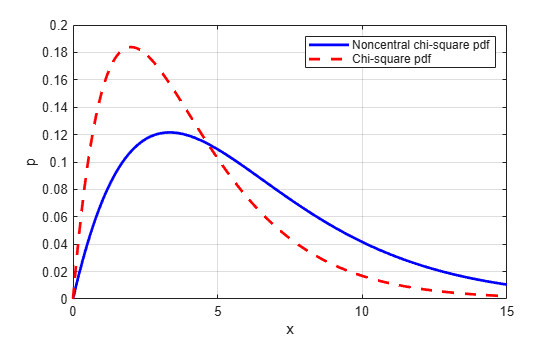ncx2pdf

Noncentral chi-square probability density function

Syntax

Y = ncx2pdf(X,V,DELTA)

Description

Y = ncx2pdf(X,V,DELTA) computes the noncentral chi-square pdf at each of the values in X using the corresponding degrees of freedom in V and positive noncentrality parameters in DELTA. Vector or matrix inputs for X, V, and DELTA must have the same size, which is also the size of Y. A scalar input for X, V, or DELTA is expanded to a constant array with the same dimensions as the other inputs.

Some texts refer to this distribution as the generalized Rayleigh, Rayleigh-Rice, or Rice distribution.

Examples

collapse all

Compute the pdf of a noncentral chi-square distribution with degrees of freedom V = 4 and noncentrality parameter DELTA = 2. For comparison, also compute the pdf of a chi-square distribution with the same degrees of freedom.

x = (0:0.1:10)';
ncx2 = ncx2pdf(x,4,2);
chi2 = chi2pdf(x,4);

Plot the pdf of the noncentral chi-square distribution on the same figure as the pdf of the chi-square distribution.

figure;
plot(x,ncx2,'b-','LineWidth',2)
hold on
plot(x,chi2,'g--','LineWidth',2)
legend('ncx2','chi2')References

 Johnson, N., and S. Kotz. Distributions in Statistics: Continuous Univariate Distributions-2. Hoboken, NJ: John Wiley & Sons, Inc., 1970, pp. 130–148.